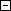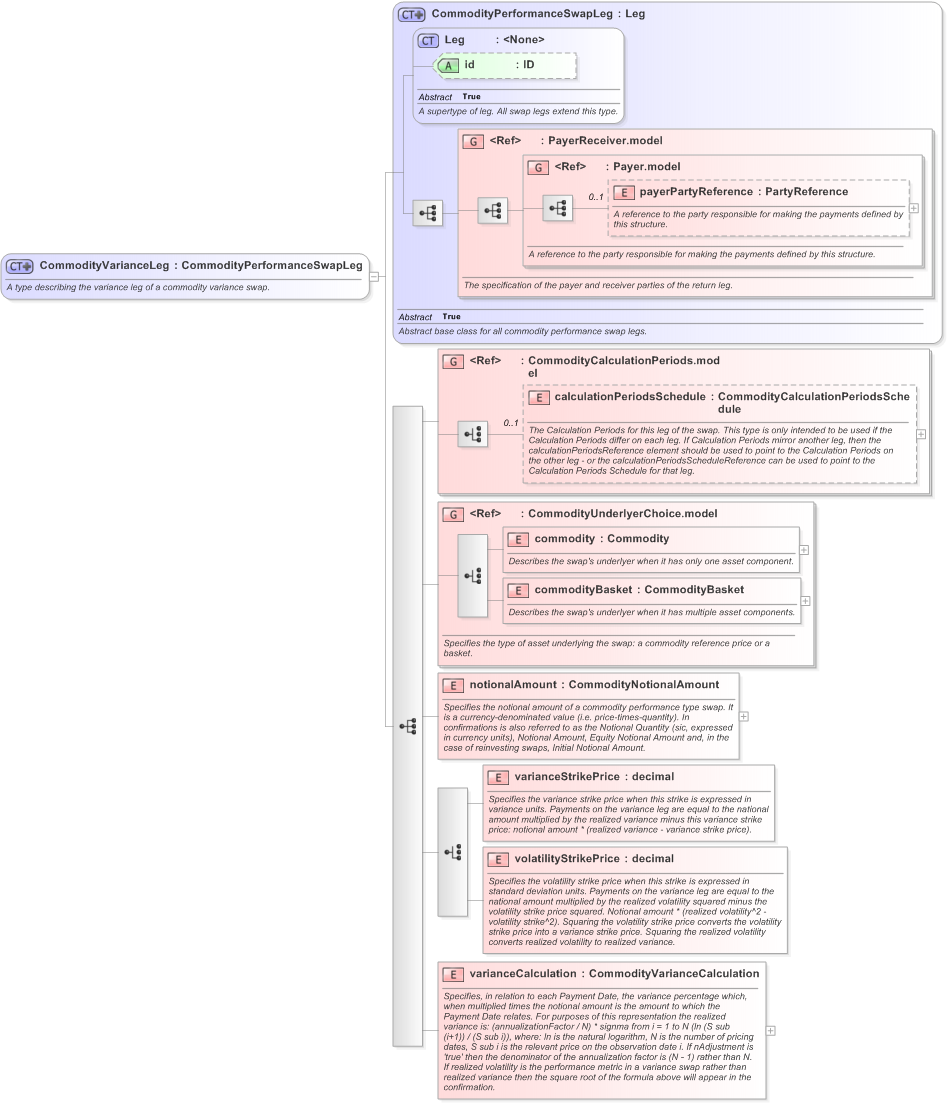Definition Type: ComplexType Name: CommodityVarianceLeg Namespace: http://www.fpml.org/FpML-5/transparency Type: nsA:CommodityPerformanceSwapLeg Containing Schema: fpml-com-5-9.xsd Abstract Documentation: A type describing the variance leg of a commodity variance swap.XSD Schema Diagram:XSD Schema Code: `````` A type describing the variance leg of a commodity variance swap. Specifies the type of asset underlying the swap: a commodity reference price or a basket. Specifies the notional amount of a commodity performance type swap. It is a currency-denominated value (i.e. price-times-quantity). In confirmations is also referred to as the Notional Quantity (sic, expressed in currency units), Notional Amount, Equity Notional Amount and, in the case of reinvesting swaps, Initial Notional Amount. Specifies the variance strike price when this strike is expressed in variance units. Payments on the variance leg are equal to the national amount multiplied by the realized variance minus this variance strike price: notional amount * (realized variance - variance strike price). Specifies the volatility strike price when this strike is expressed in standard deviation units. Payments on the variance leg are equal to the national amount multiplied by the realized volatility squared minus the volatility strike price squared. Notional amount * (realized volatility^2 - volatility strike^2). Squaring the volatility strike price converts the volatility strike price into a variance strike price. Squaring the realized volatility converts realized volatility to realized variance. Specifies, in relation to each Payment Date, the variance percentage which, when multiplied times the notional amount is the amount to which the Payment Date relates. For purposes of this representation the realized variance is: (annualizationFactor / N) * signma from i = 1 to N (ln (S sub (i+1)) / (S sub i)), where: ln is the natural logarithm, N is the number of pricing dates, S sub i is the relevant price on the observation date i. If nAdjustment is 'true' then the denominator of the annualization factor is (N - 1) rather than N. If realized volatility is the performance metric in a variance swap rather than realized variance then the square root of the formula above will appear in the confirmation. ``````Child Elements:
 Name Type Min Occurs Max Occurs payerPartyReference nsA:payerPartyReference 0 (1) calculationPeriodsSchedule nsA:calculationPeriodsSchedule 0 (1) commodity nsA:commodity (1) (1) commodityBasket nsA:commodityBasket (1) (1) notionalAmount nsA:notionalAmount (1) (1) varianceStrikePrice nsA:varianceStrikePrice (1) (1) volatilityStrikePrice nsA:volatilityStrikePrice (1) (1) varianceCalculation nsA:varianceCalculation (1) (1) nsA:PayerReceiver.model (1) (1) nsA:Payer.model (1) (1) nsA:CommodityCalculationPeriods.model (1) (1) nsA:CommodityUnderlyerChoice.model (1) (1)Child Attributes:
 Name Type Default Value Use id nsA:id (Optional)Derivation Tree: nsA:LegnsA:CommodityPerformanceSwapLeg CommodityVarianceLegReferences: nsA:commodityVarianceLegComments: Please enable JavaScript to view the comments powered by Disqus. blog comments powered by Disqus